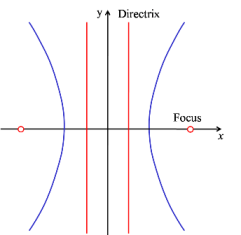The Hyperbola is a plane curve. The Hyperbola is a conic section.

The Hyperbola is defined as the path of a point (locus) which moves such that its distance from a fixed point (called the focus) is greater than its distance from a fixed line (called the directrix).

The ratio of the distance to the focus to the distance to the directrix is called the eccentricity normally denoted by e.

For an hyperbola therefore e > 1.

When the directrix is $x=\frac{a}{e}$ and the focus is ( ae, 0) the hyperbola will have the equation in Cartesian Coordinates $\frac{x^2}{a^2}-\frac{y^2}{b^2}=1$ where $b^2=a^2(e^2-1)$

One of the Named Curves on this site.